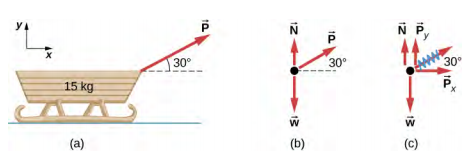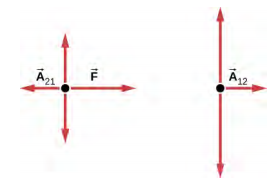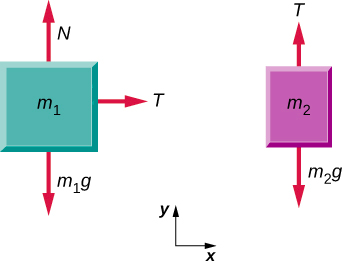# 5.15: Drawing Free-Body Diagrams

•• OpenStax
• OpenStax
$$\newcommand{\vecs}{\overset { \rightharpoonup} {\mathbf{#1}} }$$ $$\newcommand{\vecd}{\overset{-\!-\!\rightharpoonup}{\vphantom{a}\smash {#1}}}$$$$\newcommand{\id}{\mathrm{id}}$$ $$\newcommand{\Span}{\mathrm{span}}$$ $$\newcommand{\kernel}{\mathrm{null}\,}$$ $$\newcommand{\range}{\mathrm{range}\,}$$ $$\newcommand{\RealPart}{\mathrm{Re}}$$ $$\newcommand{\ImaginaryPart}{\mathrm{Im}}$$ $$\newcommand{\Argument}{\mathrm{Arg}}$$ $$\newcommand{\norm}{\| #1 \|}$$ $$\newcommand{\inner}{\langle #1, #2 \rangle}$$ $$\newcommand{\Span}{\mathrm{span}}$$ $$\newcommand{\id}{\mathrm{id}}$$ $$\newcommand{\Span}{\mathrm{span}}$$ $$\newcommand{\kernel}{\mathrm{null}\,}$$ $$\newcommand{\range}{\mathrm{range}\,}$$ $$\newcommand{\RealPart}{\mathrm{Re}}$$ $$\newcommand{\ImaginaryPart}{\mathrm{Im}}$$ $$\newcommand{\Argument}{\mathrm{Arg}}$$ $$\newcommand{\norm}{\| #1 \|}$$ $$\newcommand{\inner}{\langle #1, #2 \rangle}$$ $$\newcommand{\Span}{\mathrm{span}}$$$$\newcommand{\AA}{\unicode[.8,0]{x212B}}$$

##### Learning Objectives
• Explain the rules for drawing a free-body diagram
• Construct free-body diagrams for different situations

The first step in describing and analyzing most phenomena in physics involves the careful drawing of a free-body diagram. Free-body diagrams have been used in examples throughout this chapter. Remember that a free-body diagram must only include the external forces acting on the body of interest. Once we have drawn an accurate free-body diagram, we can apply Newton’s first law if the body is in equilibrium (balanced forces; that is, $$F_{net} = 0$$) or Newton’s second law if the body is accelerating (unbalanced force; that is, $$F_{net} \neq 0$$).

In Forces, we gave a brief problem-solving strategy to help you understand free-body diagrams. Here, we add some details to the strategy that will help you in constructing these diagrams.

##### Problem-Solving Strategy: Constructing Free-Body Diagrams

Observe the following rules when constructing a free-body diagram:

1. Draw the object under consideration; it does not have to be artistic. At first, you may want to draw a circle around the object of interest to be sure you focus on labeling the forces acting on the object. If you are treating the object as a particle (no size or shape and no rotation), represent the object as a point. We often place this point at the origin of an xy-coordinate system.
2. Include all forces that act on the object, representing these forces as vectors. Consider the types of forces described in Common Forces—normal force, friction, tension, and spring force—as well as weight and applied force. Do not include the net force on the object. With the exception of gravity, all of the forces we have discussed require direct contact with the object. However, forces that the object exerts on its environment must not be included. We never include both forces of an action-reaction pair.
3. Convert the free-body diagram into a more detailed diagram showing the x- and y-components of a given force (this is often helpful when solving a problem using Newton’s first or second law). In this case, place a squiggly line through the original vector to show that it is no longer in play—it has been replaced by its x- and y-components.
4. If there are two or more objects, or bodies, in the problem, draw a separate free-body diagram for each object.

Note: If there is acceleration, we do not directly include it in the free-body diagram; however, it may help to indicate acceleration outside the free-body diagram. You can label it in a different color to indicate that it is separate from the free-body diagram.

Let’s apply the problem-solving strategy in drawing a free-body diagram for a sled. In Figure $$\PageIndex{1a}$$, a sled is pulled by force $$\vec{P}$$ at an angle of 30°. In part (b), we show a free-body diagram for this situation, as described by steps 1 and 2 of the problem-solving strategy. In part (c), we show all forces in terms of their x- and y-components, in keeping with step 3.Figure $$\PageIndex{1}$$: (a) A moving sled is shown as (b) a free-body diagram and (c) a free-body diagram with force components.
##### Example $$\PageIndex{1}$$: Two Blocks on an Inclined Plane

Construct the free-body diagram for object A and object B in Figure $$\PageIndex{1}$$.

Strategy

We follow the four steps listed in the problem-solving strategy.

Solution

We start by creating a diagram for the first object of interest. In Figure $$\PageIndex{2a}$$, object A is isolated (circled) and represented by a dot.Figure $$\PageIndex{2}$$: (a) The free-body diagram for isolated object A. (b) The free-body diagram for isolated object B. Comparing the two drawings, we see that friction acts in the opposite direction in the two figures. Because object A experiences a force that tends to pull it to the right, friction must act to the left. Because object B experiences a component of its weight that pulls it to the left, down the incline, the friction force must oppose it and act up the ramp. Friction always acts opposite the intended direction of motion.

We now include any force that acts on the body. Here, no applied force is present. The weight of the object acts as a force pointing vertically downward, and the presence of the cord indicates a force of tension pointing away from the object. Object A has one interface and hence experiences a normal force, directed away from the interface. The source of this force is object B, and this normal force is labeled accordingly. Since object B has a tendency to slide down, object A has a tendency to slide up with respect to the interface, so the friction fBA is directed downward parallel to the inclined plane.

As noted in step 4 of the problem-solving strategy, we then construct the free-body diagram in Figure 5.32(b) using the same approach. Object B experiences two normal forces and two friction forces due to the presence of two contact surfaces. The interface with the inclined plane exerts external forces of NB and fB, and the interface with object B exerts the normal force NAB and friction fAB; NAB is directed away from object B, and fAB is opposing the tendency of the relative motion of object B with respect to object A.

Significance

The object under consideration in each part of this problem was circled in gray. When you are first learning how to draw free-body diagrams, you will find it helpful to circle the object before deciding what forces are acting on that particular object. This focuses your attention, preventing you from considering forces that are not acting on the body

##### Example $$\PageIndex{2}$$: Two Blocks in Contact

A force is applied to two blocks in contact, as shown.

Strategy

Draw a free-body diagram for each block. Be sure to consider Newton’s third law at the interface where the two blocks touch.Solution

##Significance

$$\vec{A}_{21}$$ is the action force of block 2 on block 1. $$\vec{A}_{12}$$ is the reaction force of block 1 on block 2. We use these free-body diagrams in Applications of Newton’s Laws.

##### Example $$\PageIndex{3}$$: Block on the Table (Coupled Blocks)

A block rests on the table, as shown. A light rope is attached to it and runs over a pulley. The other end of the rope is attached to a second block. The two blocks are said to be coupled. Block m2 exerts a force due to its weight, which causes the system (two blocks and a string) to accelerate.

Strategy

We assume that the string has no mass so that we do not have to consider it as a separate object. Draw a free-body diagram for each block.SolutionSignificance

Each block accelerates (notice the labels shown for $$\vec{a}_{1}$$ and $$\vec{a}_{2}$$); however, assuming the string remains taut, they accelerate at the same rate. Thus, we have |$$\vec{a}_{1}$$| = |$$\vec{a}_{2}$$|. If we were to continue solving the problem, we could simply call the acceleration $$\vec{a}$$. Also, we use two free-body diagrams because we are usually finding tension T, which may require us to use a system of two equations in this type of problem. The tension is the same on both m1 and m2.

##### Exercise $$\PageIndex{1}$$
1. Draw the free-body diagram for the situation shown.
2. Redraw it showing components; use x-axes parallel to the two ramps.##### Simulation

View this simulation to predict, qualitatively, how an external force will affect the speed and direction of an object’s motion. Explain the effects with the help of a free-body diagram. Use free-body diagrams to draw position, velocity, acceleration, and force graphs, and vice versa. Explain how the graphs relate to one another. Given a scenario or a graph, sketch all four graphs.

This page titled 5.15: Drawing Free-Body Diagrams is shared under a CC BY license and was authored, remixed, and/or curated by OpenStax.